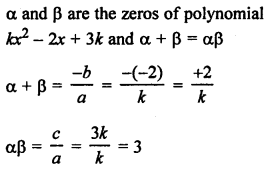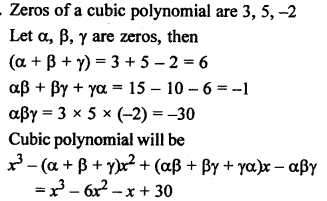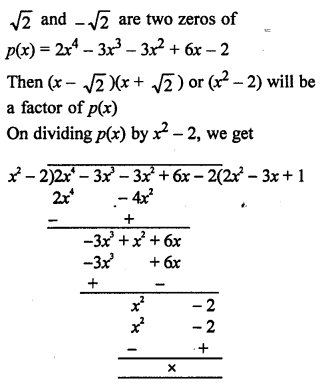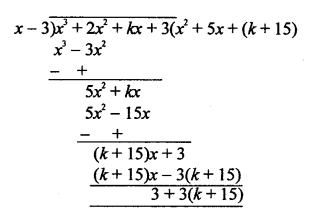# RS Aggarwal Solutions Class 10 Chapter 2 Polynomials Test Yourself

In this chapter, we provide RS Aggarwal Solutions for Class 10 Chapter 2 Polynomials Test Yourself Maths for English medium students, Which will very helpful for every student in their exams. Students can download the latest RS Aggarwal Solutions for Class 10 Chapter 2 Polynomials Test Yourself Maths pdf, free RS Aggarwal Solutions Class 10 Chapter 2 Polynomials Test Yourself Maths book pdf download. Now you will get step by step solution to each question.

#### RS Aggarwal Class 10 Solutions Chapter 2 Polynomials Test Yourself

Question 1.
Solution:
(b) Polynomial is x2 – 2x – 3
=> x2 – 3x + x – 3
= x(x – 3) + 1(x – 3)
= (x – 3) (x + 1)
Either x – 3 = 0, then x = 3
or x + 1 = 0, then x = -1
Zeros are 3, -1

Question 2.
Solution:
(a) α, β, γ are the zeros of Polynomial is x3 – 6x2 – x + 30
Here, a = 1, b = -6, c = -1, d = 30
αβ + βγ + γα = ca = −11 = -1

Question 3.
Solution:
(c)Question 4.
Solution:
(c) Let α and β be the zeros of the polynomial 4x2 – 8kx + 9Question 5.
Solution:Either x + 15 = 0, then x = -15
or x – 13 = 0, then x = 13
Zeros are 13, -15

Question 6.
Solution:
The polynomial is (a2 + 9) x2 + 13x + 6aQuestion 7.
Solution:
Zeros are 2 and -5
Sum of zeros = 2 + (-5) = 2 – 5 = -3
and product of zeros = 2 x (-5) = -10
Now polynomial will be
x2 – (Sum of zeros) x + Product of zeros
= x2 – (-3)x + (-10)
= x2 + 3x – 10

Question 8.
Solution:
(a – b), a, (a + b) are the zeros of the polynomial x3 – 3x2 + x + 1
Here, a = 1, b = -3, c = 1, d = 1
Now, sum of zeros = −ba = −(−3)1 = 3
a – b + a + a + b = 3Question 9.
Solution:
Let f(x) = x3 + 4x2 – 3x – 18
If 2 is its zero, then it will satisfy it
Now, (x – 2) is a factor Dividing by (x – 2)
Hence, x = 2 is a zero of f(x)Question 10.
Solution:
Sum of zeros = -5
and product of zeros = 6
x2 – (Sum of zeros) x + Product of zeros
= x2 – (-5) x + 6
= x2 + 5x + 6

Question 11.
Solution:Question 12.
Solution:
p(x) = x3 + 3x2 – 5x + 4
g(x) = x – 2
Let x – 2 = 0, then x = 2
Remainder = p(2) = (2)3 + 3(2)2 – 5(2) + 4 = 8 + 12 – 10 + 4 = 14

Question 13.
Solution:
f(x) = x3 + 4x2 + x – 6
and g(x) = x + 2
Let x + 2 = 0, then x = -2
f(-2) = (-2)3 + 4(-2)2 + (-2) – 6 = -8 + 16 – 2 – 6 = 0
Remainder is zero, x + 2 is a factor of f(x)

Question 14.
Solution:Question 15.
Solution:Question 16.
Solution:
f(x) = x4 + 4x2 + 6
=> (x2)2 + 4(x2) + 6 = y2 + 4y + 6 (Let x2 = y)
Let α, β be the zeros of y2 + 4y + 6
Sum of zeros = -4
and product of zeros = 6
But there are no factors of 6 whose sum is -4 {Factors of 6 = 1 x 6 and 2 x 3}
Hence, f(x) Has no zero (real).

Question 17.
Solution:
3 is one zero of p(x) = x3 – 6x2 + 11x – 6
(x – 3) is a factor of p(x)
Dividing, we getQuestion 18.
Solution:Question 19.
Solution:
p(x) = 3x4 + 5x3 – 7x2 + 2x + 2
Dividing by x2 + 3x + 1,
we get,
Quotient = 3x2 – 4x + 2Question 20.
Solution:
Let p(x) = x3 + 2x2 + kx + 3
g(x) = x – 3
and r(x) = 21
Dividing p(x) by g(x), we getBut remainder = 21
3 + 3k + 45 = 21
3k = 21 – 45 – 3
=> 3k = 21 – 48 = -27
k = -9
Second method:
x – 3 is a factor of p(x) : x = 3
Substituting the value of x in p(x)
p(3) = 33 + 2 x 32 + k x 3 + 3
= 27 + 18 + 3k + 3
48 + 3k = 21
=> 3k = -48 + 21 = -27
k = -9
Hence, k = -9

All Chapter RS Aggarwal Solutions For Class 10 Maths

—————————————————————————–

All Subject NCERT Exemplar Problems Solutions For Class10

All Subject NCERT Solutions For Class 10

*************************************************

I think you got complete solutions for this chapter. If You have any queries regarding this chapter, please comment on the below section our subject teacher will answer you. We tried our best to give complete solutions so you got good marks in your exam.

If these solutions have helped you, you can also share rsaggarwalsolutions.in to your friends.